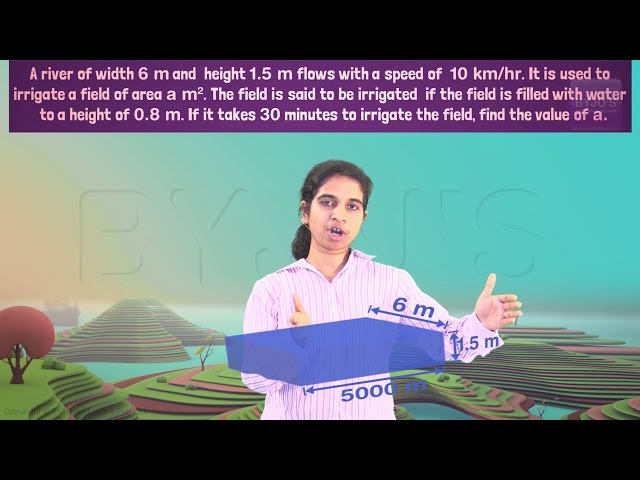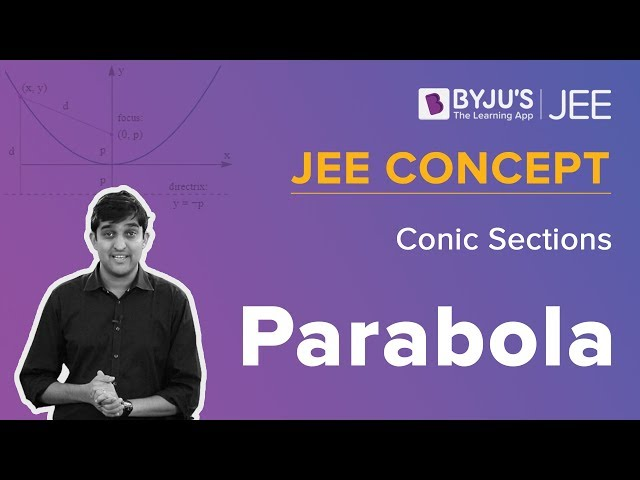Win up to 100% scholarship on Aakash BYJU'S JEE/NEET courses with ABNAT Win up to 100% scholarship on Aakash BYJU'S JEE/NEET courses with ABNAT

# JEE Main 2018 Maths Paper With Solutions Shift 1 Jan 10

JEE Main 2018 Maths paper January 10 Shift 1 solutions are available on this page. Students are recommended to revise these solutions so that they can increase their speed and accuracy. These solutions will help them to understand the difficulty level of questions from each chapter. Students can easily download them in PDF format for free.
January 10 Shift 1 – Maths
1. If the tangent at (1, 7) to the curve x2 = y-6 touches the circle x2+y2+16x+12y+c = 0, then the value of c is :
a) 85
b) 95
c) 195
d) 185

Equation of tangent at (1, 7) to x2 = y-6 is 2x-y = -5.

It touches circle x2+y2+16x+12y+c = 0.

Hence length of perpendicular from centre (-8, -6) to tangent equals radius of circle.

d =

$$\begin{array}{l}\left | \frac{-16+6+5}{\sqrt{2^{2}+(-1)^{2}}} \right |= \sqrt{5}\end{array}$$

$$\begin{array}{l}\sqrt{64+36-c}\end{array}$$

√(100-c) = √5

So c = 95

2. If L1 is the line of intersection of the planes 2x-2y+3z-2 = 0, x-y+z+1 = 0 and L2 is the line of intersection of the planes x+2y-z-3 = 0, 3x-y+2z-1 = 0, then the distance of the origin from the plane, containing the lines L1 and L2 is :
a) 1/2√2
b) 1/√2
c) 1/4√2
d) 1/3√2

L1 =

$$\begin{array}{l}\begin{vmatrix} l &m &n \\ 2& -2 & 3\\ 1 & -1 & 1 \end{vmatrix}\end{array}$$

= l+m (drs of line L1)

L2 =

$$\begin{array}{l}\begin{vmatrix} l &m &n \\ 1& 2 & -1\\ 3 & -1 & 2 \end{vmatrix}\end{array}$$

= 3l-5m-7n (drs of line L2)

Normal plane containing line L1 and L2 =

$$\begin{array}{l}\begin{vmatrix} l &m &n \\ 1& 1 & 0\\ 3 & 5 & -7 \end{vmatrix}\end{array}$$

= 7l-7m-8n

For one point of line L1

2x-2y+3z-2 = 0

x-y+z+1 = 0

Put x = 0, and solving above equations we get (0, 5, 4)

So the equation of the plane is -7(x-0)+7(y-5)-8(z-4) = 0

7x-7y+8z+3 = 0

Distance =

$$\begin{array}{l}\left | \frac{7\times 0-7\times 0+8\times 0+3}{\sqrt{7^{2}+7^{2}+8^{2}}} \right |\end{array}$$

= 3/√162

= 1/3√2

3. If α and β ∈ C are the distinct roots of the equation x2-x+1 = 0, then α101107 is equal to:
a) 1
b) 2
c) -1
d) 0

x2-x+1 = 0

x =

$$\begin{array}{l}\frac{1\pm \sqrt{1-4\times 1\times 1}}{2\times 1}\end{array}$$

= (1±i√3)/2

= (1+i√3)/2 , (1-i√3)/2

= -(-1-i√3)/2, -(-1+i√3)/2

= -ω2, -ω

α= -ω2

β = -ω

α101107 = (-ω2)101+(-ω)107

= -(ω202+ ω107)

= -[(ω3)67 ω+ (ω3)35 ω2]

= -[ω +ω2]

= 1

4. Tangents are drawn to the hyperbola 4x2– y2 = 36 at the points P and Q. If these tangents intersect at the point T(0, 3) then the area (in sq. units) of ar(PTQ) is :
a) 60√3
b) 36√5
c) 45√5
d) 54√3

Given equation of hyperbola is 4x2-y2 = 36

(x2/9)-(y2/36) = 1

a2 = 9

b2 = 36

From T(0, 3) tangents are drawn to hyperbola at P and Q.

Hence equation of Chord of contact PQ is

(x(0)/9) – (y(3)/36) = 1

y = -12

(x2/9)-(144/36) = 1

x2 = 45

x = ±3√5

Hence P = (3√5, -12) and Q = (-3√5, -12)

Hence ar(PQT) is

$$\begin{array}{l}\frac{1}{2}\begin{vmatrix} 3\sqrt{5} & -12 & 1\\ -3\sqrt{5} & -12&1 \\ 0& 3 & 1 \end{vmatrix} = 45\sqrt{5}\end{array}$$

5. If the curves y2 = 6x, 9x2 + by2 = 16 intersect each other at right angles, then the value of b is :
a) 4
b) 9/2
c) 6
d) 7/2

Given y2 = 6x

Differentiating

2y(dy/dx) = 6

(dy/dx) = 3/y

Given 9x2 + by2 = 16

Differentiating

18x+2by(dy/dx) = 0

(dy/dx) = -18x/2by

(dy/dx) = -9x/by

Let the intersection point be (x1, y1)

m1 = 3/y1

m2 = -9x1 /by1

Since the curves intersect at right angles, m1m2 = -1

(3/y1)( -9x1 /by1) = -1

27x1 = by12 [Since (x1, y1) lies on y2 = 6x y12 = 6x1]

27x1 = b(6x1)

b = 9/2

6. If the system of linear equations :

x+ky+3z = 0

3x+ky-2z = 0

2x+4y-3z = 0

has a non-zero solution (x, y, z), then xz/y2 is equal to :

a) -30
b) 30
c) -10
d) 10

Given system of equations have non zero solutions.

So, Δ =

$$\begin{array}{l}\begin{vmatrix} 1 & k &3 \\ 3 & k & -2\\ 2&4 & -3 \end{vmatrix}= 0\end{array}$$

1(-3k+8)-k(-9+4)+3(12-2k) = 0

-3k+8+5k+36-6k = 0

4k = 44

k = 44/4 = 11

Now the given equations become

x+11y+3z = 0

3x+11y-2z = 0

2x+4y-3z = 0

x/(-22-33) = y/(-(-2-9) = z/(11-33)

x/-55 = y/11 = x/-22

x/5 = y/-1 = z/2 = L (let)

xz/y2 = (5L)(2L)/(-L2) = 10

7. Let S = {x ∈R: x ≥ 0 and 2|√x-3|+√x(√x-6)+6 = 0}. Then S:
a) contains exactly two elements.
b) contains exactly four elements.
c) is an empty set.
d) contains exactly one element.

2|√x-3|+√x(√x-6)+6 = 0

2√x-6+x-6√x+6 = 0 if √x >3

x-4√x = 0

√x = 0 or 4

√x = 4

Also 2(3-√x)+√x(√x-6)+6 = 0 if √x<3

6-2√x+x-6√x+6 = 0

x-8√x+12 = 0

(√x)2-6√x-2√x+12 = 0

(√x-2)(√x-6) = 0

√x = 2, 6

x = 4

8. If sum of all the solutions of the equation 8cos x(cos ((π/6)+x). cos ((π/6)-(x))-(1/2)) = 1 in [0, π] is kπ, then k is equal to :
a) 8/9
b) 20/9
c) 2/3
d) 13/9

8cos x(cos ((π/6)+x). cos ((π/6)-(1/2))-(1/2)) = 1

8.cos x[(cos (π/3)+ cos 2x-2)/2] = 1

4 cosx(cos 2x-(1/2)) = 1

4 cosx(2cos2x-(3/2)) = 1

8cos3x-6cosx-1 = 0

2(4cos3x-3cosx)-1 = 0

2cos3x-1 = 0

cos 3x = 1/2 = cos(π/3)

3x = 2nπ ±π/3

x = (2nπ /3) ±(π/9)

= 7π/9, 5π/9, π/9 in [0, π]

k = 13/9

9. A bag contains 4 red and 6 black balls. A ball is drawn at random from the bag, its colour is observed and this ball along with two additional balls of the same colour are returned to the bag. If now a ball is drawn at random from the bag, then the probability that this drawn ball is red, is :
a) 1/5
b) 3/4
c) 3/10
d) 2/5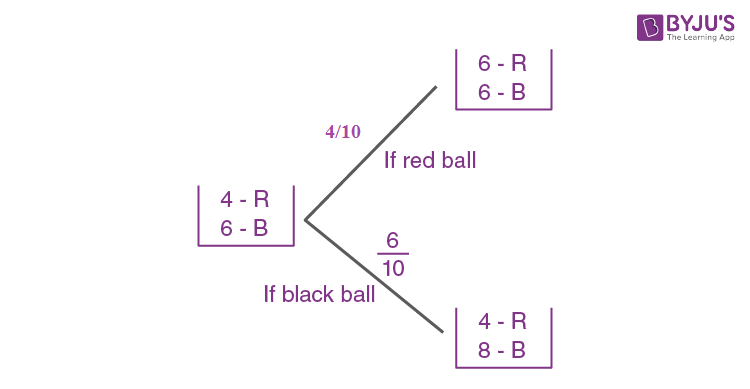From total Probability Theorem

P (R) = (4/10)×(1/2)+(6/10) × (4/12)

= (1/5)+(1/5)

= 2/5

10. Let f(x) = x2+(1/x2) and g(x) = x-(1/x), x∈ R-{-1,0,1}. If h(x) = f(x)/g(x), then the local minimum value of h(x) is:
a) -2√2
b) 2√2
c) 3
d) -3

f(x) = x2+(1/x2)

= (x-(1/x))2+2

g(x) = x-(1/x)

Put x-(1/x) = t

h(x) = f(x)/g(x)

Let h(x) = k(t)

= (t2+2)/t

= t+2/t

Differentiating

k’(t) = 1-2/t2

For minima or maxima, 1-2/t2 = 0

t2 = 2

t = ±√2

k’’(t) = 4/t3

At t = √2, k’’(t) = 2/√2

At t = -√2, k’’(t) = -2/√2

At t = √2. k’’(t) is positive.

So it is a minima.

h(x) = t+2/t

= √2+2/√2

= 2√2

11. Two sets A and B are as under :

A = {(a,b) ∈R×R:|a-5| < 1 and |b-5| < 1}

B = {(a,b) ∈R×R: 4(a-6)2+9(b-5)2 ≤ 36}. Then:

a) A⋂B = phi(an empty set)
b) neither A⊂B nor B⊂A
c) B⊂A
d) A⊂B

A = {(a,b) ∈R×R:|a-5| < 1 and |b-5| < 1}

-1<a-5<1

4<a<6

4<b<6

B = {(a,b) ∈R×R

4(a-6)2+9(b-5)2 ≤ 36

((a-6)2 /9 )+((b-5)2 /4) ≤ 1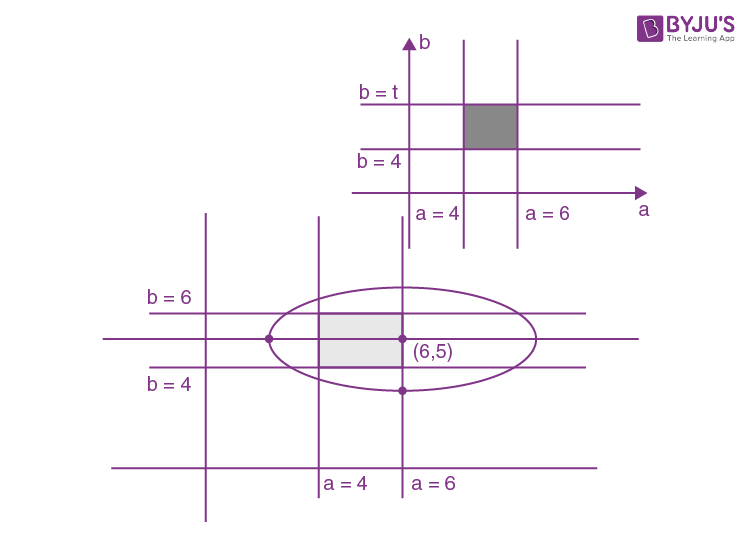12. The Boolean expression : ~(p ˅q) ˅(~p˄q) is equivalent to:
a) q
b) ~q
c) ~p
d) p

 p (1) q (2) ~p (3) (p ˅q) (4) ~(p ˅q) (5) ~p˄q (6) ~(p ˅q) ˅(~p˄q) T T F T F F F T F F T F F F F T T T F T T F F T F T F T

Entries in column (3) and (7) are identical.

13. Tangent and normal are drawn at P(16, 16) on the parabola y2 = 16x, which intersect the axis of the parabola at A and B, respectively. If C is the centre of the circle through the points P, A and B and ∠CPB = θ, then a value of tan θ is :
a) 3
b) 4/3
c) 1/2
d) 2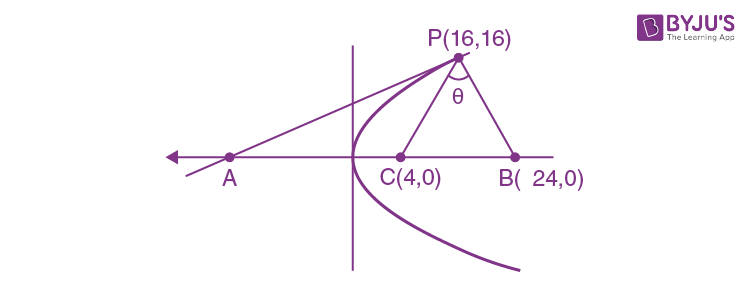Tangent and normal are drawn at P (16, 16) on y2 = 16x

y2 = 16x

Slope of tangent dy/dx = 16/2y

= 8/y

(dy/dx)(16,16) = 1/2

Equation of the tangent is

y-16 = (1/2)(x-16)

2y-32 = x-16

x-2y+16 = 0 ..(1)

Equation of normal

y-16 = -2(x-16)

2x+y-48 = 0 ..(2)

Tangent & normal intersect the axis of parabola

A(-16,0)

B(24,0)

Slope of AP×slope of PB

[(16-0)/(16+16)][(16-0)/(16-24)] = -1

So AB is the diameter of circle with centre C(4,0)

mPC = (16-0)/(16-4) = 16/12 = 4/3

mPB = (16-0)/(16-24) = 16/-8 = -2

tan = m1 -m2/(1+m1m2)

= (4/3)+2)/(1+(4/3)×2)

= 2

14. If
$$\begin{array}{l}\begin{vmatrix} x-4 & 2x &2x \\ 2x& x-4 & 2x\\ 2x& 2x & x-4 \end{vmatrix}\end{array}$$
= (A+Bx)(x-A)2 then the ordered pair (A, B) is equal to :

a) (-4, 5)
b) (4, 5)
c) (-4, -5)
d) (-4, 3)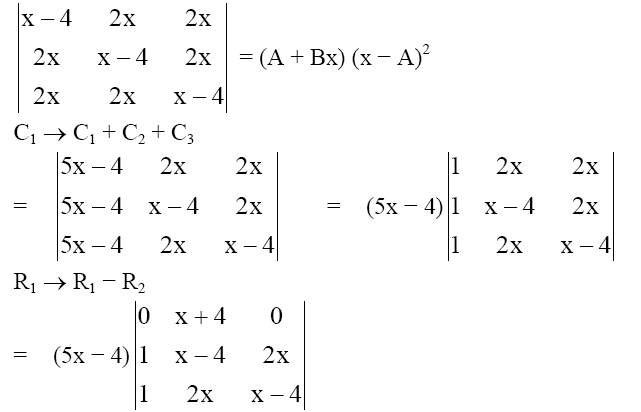= (5x-4)(-1)(x+4)(x-4-2x)

= -1(5x-4)(x+4)(-x-4)

= (5x-4)(x+4)(x+4)2

= (-4+5x)(x-(-4))2

Hence A = -4, B = 5

15. The sum of the coefficients of all odd degree terms in the expansion of (x+√(x3-1))5+(x-√(x3-1))5, (x>) is :
a) 1
b) 2
c) -1
d) 0

(a+b)n+(a-b)n = 2(nC0an + nC2an-2b2+ nC4an-4b4…)

(x+√(x3-1))5+(x-√(x3-1))5, (x>)

= 2(5C0x5+5C2x3(√(x3-1))2+5C4x(√(x3-1))4)

= 2(x5+10x6-10x3+5x7-10x4+5x)

Sum of the coefficient of odd term is given by

2(1-10+5+5)

= 2

16. Let a1, a2, a3,..a49 be in A.P such that Ʃk=012 a4k+1 = 416 and a9+a43 = 66. If a12+a22+…+a172 = 140m, then m is equal to:
a) 34
b) 33
c) 66
d) 68

a1, a2, a3,..a49 are in A.P

Let A be the first term and D is the common difference.

a9+a43 = 66

A+8D+A+42D = 66

2A+50D = 66

A+25D = 33

a26 = 33 ..(i)

Ʃk=012 a4k+1 = 416

a1+a5+a9+…+a49 = 416

13A+312D = 416

A+24D = 23

a25 = 32 ..(ii)

From (i) and (ii), a26-a25 = D = 1

Also A+25D = 33

So A = 8

a12+a22+…+a172 = 82+92+102+…+242

Ʃr=124 r2– Ʃr=17 r2 = 140m

(24×25×49/6)-(7× 8 ×15/6) = 140m

4900-140 = 140m

m = 34

17. A straight line through a fixed point (2, 3) intersects the coordinate axes at distinct points P and Q. If O is the origin and the rectangle OPRQ is completed, then the locus of R is :
a) 3x+2y = xy
b) 3x+2y = 6xy
c) 3x+2y = 6
d) 2x+3y = xy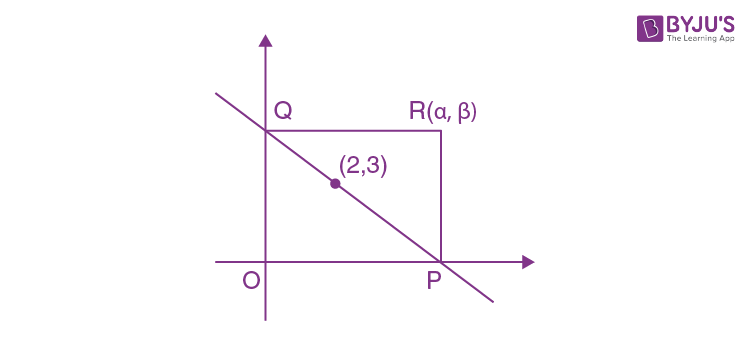line y-3 = m(x-2)

y-3 = mx-2m

P = ((2m-3)/m, 0)

Q = (0, 3-2m)

Let R(α, β)

So α= ((2m-3)/m, 0) and β = 3-2m

m = 3/(2-α) and m = (3-β)/2

3/(2-α) = (3-β)/2

6 = 6-2β-3α+αβ

Locus of R (α,β) is 3x+2y = xy

18. The value of
$$\begin{array}{l}\int_{\frac{-\pi }{2}}^{\frac{\pi }{2}}\frac{\sin ^{2}x}{1+2^{x}}dx\end{array}$$
is:

a) 4π
b) π/4
c) π/8
d) π/2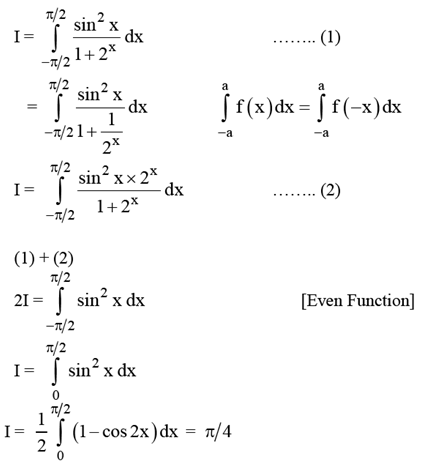19. Let g(x) = cos x2, f(x) = √x and α, β (α< β) be the roots of the quadratic equation 18x2-9πx+π2 = 0. Then the area in sq.units) bounded by the curve y = (gof)(x) and the lines x = αand x = β and y =0 is:
a) (1/2)(√3-√2)
b) (1/2)(√2-1)
c) (1/2)(√3-1)
d) (1/2)(√3+1)

18x2-9πx+π2 = 0

x = π/6, π/3 (so α= π/6, β= π/3)

y = (gof)(x)

= g(f(x))

= g(√x)

= cos x

A =

$$\begin{array}{l}\int_{\frac{\pi }{6}}^{\frac{\pi }{3}}\cos x dx = [\sin x]^{\frac{\pi }{3}}_{\frac{\pi }{6}}\end{array}$$

= (√3/2)-(1/2)

= (√3-1)/2

20. For each t ∈ R, let [t] be the greatest integer less than or equal to t. Then

$$\begin{array}{l}\lim_{x\to0+}x([\frac{1}{x}]+[\frac{2}{x}]+…[\frac{15}{x}])\end{array}$$

a) is equal to 120
b) does not exist (in R)
c) is equal to 0
d) is equal to 15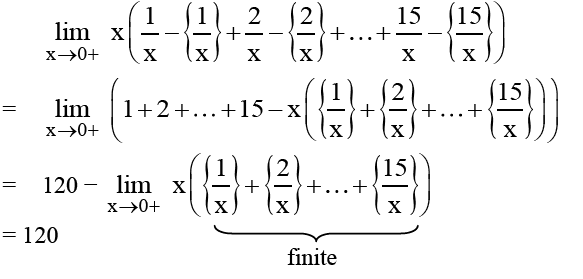21. If ∑9i=1 (xi-5) = 9 and ∑9i=1 (xi-5)2 = 45, then the standard deviation of the 9 items x1, x2,…x9 is:
a) 2
b) 3
c) 9
d) 4

Variance = (1/n) Ʃi=1n xi2-[(1/n) Ʃi=1n xi]2

= (1/9)×45-((1/9)×9)2

= 5-1

= 4

S.D = √4 = 2

22. The integral
$$\begin{array}{l}\int \frac{\sin ^{2}x\cos ^{2}x}{\sin ^{5}x+\cos ^{3}x\sin ^{2}x+\sin ^{3}x\cos ^{2}x+\cos ^{5}x}dx\end{array}$$
is equal to:

where C is the constant of integration.

a) 1/(1+cot3x) + C
b) -1/(1+cot3x) + C
c) 1/3(1+tan3x) + C
d) -1/3(1+tan3x) + C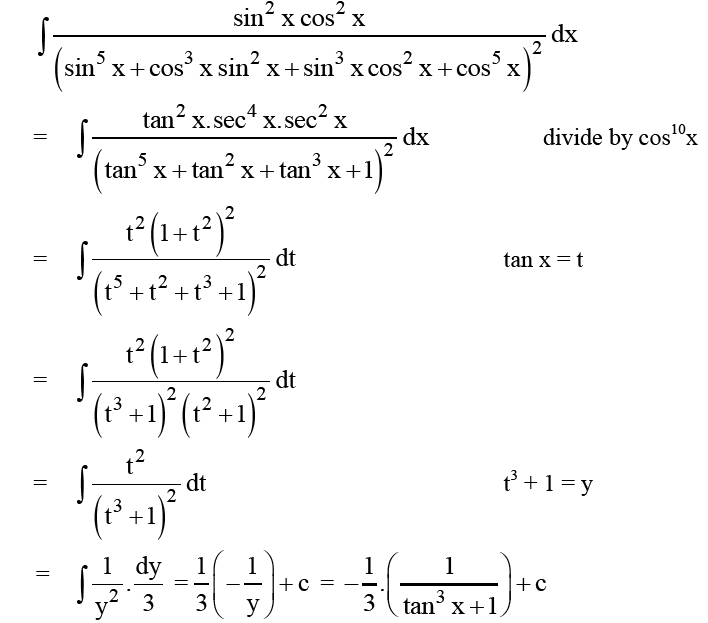23. Let S = {t∈R: f(x) = |x-π|(e|x|-1)sin x is not differentiable at t}. Then the set S is equal to:
a) {π}
b) {0,π}
c) (an empty set)
d) {0}

f(x) = |x-π|(e|x|-1) sinx

Obviously differentiable at x = 0

Check at x = π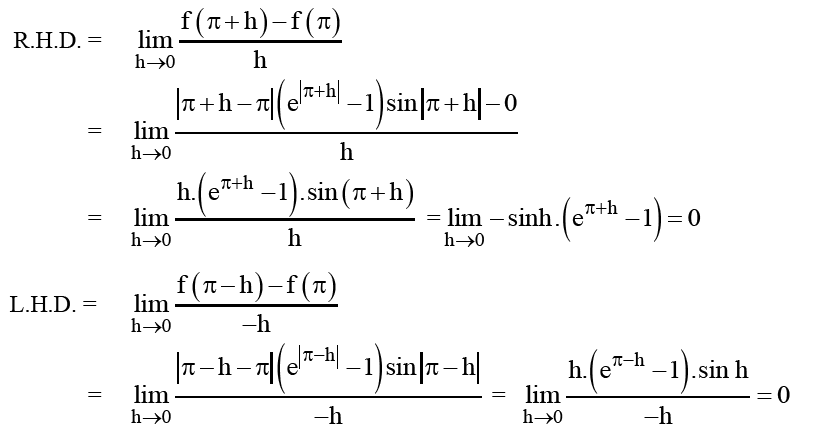So, differentiable at x = π.

24. Let y = y(x) be the solution of the differential equation sin x(dy/dx)+y cosx = 4x, x∈(0,π). If y(π/2) = 0, then y(π/6) is equal to:
a) (-8/9)π2
b) (-4/9)π2
c) (4/9√3)π2
d) (-8/9√3)π2

(dy/dx)+y cotx = 4x/sin x

IF = e∫cot xdx = elog|sin x| = sin x as x∈(0,π)

y sin x = c+∫4x dx

= c+2x2

As y(π/2) = 0 0.sin(π/2) = c+2(π/2)2

c = -(π2/2)

So y sin x = 2x2-(π2/2)

Put x = π/6

y sin π/6 = 2(π/6)2-(π2/2)

y(1/2) = (π2/18)- (π2/2)

= (-8π2/9)

= (-8/9)π2

25. Let u be a vector coplanar with the vectors

$$\begin{array}{l}\vec{a}= 2\hat{i}+3\hat{j}-\hat{k}\end{array}$$

and

$$\begin{array}{l}\vec{b}= \hat{j}+\hat{k}\end{array}$$
. If vector u is perpendicular to vector a and
$$\begin{array}{l}\vec{u}.\vec{b}= 24\end{array}$$
, then u2 is equal to:

a) 256
b) 84
c) 336
d) 315

$$\begin{array}{l}\vec{u}= u_{1}\hat{i}+u_{2}\hat{j}+u_{3}\hat{k}\end{array}$$

As coplanar

$$\begin{array}{l}\begin{vmatrix} u_{1} & u_{2} &u_{3} \\ 2& 3 & -1\\ 0&1 & 1 \end{vmatrix}=0\end{array}$$

4u1-2u2+2u3 = 0

2u1-u2+u3 = 0 ..(1)

$$\begin{array}{l}\vec{u}.\vec{a}=0\end{array}$$

2u1-3u2-u3 = 0 ..(2)

$$\begin{array}{l}\vec{u}.\vec{b}=0\end{array}$$

u2+u3= 24 ..(3)

Solving u1 = -4, u2 = 8, u3 = 16

$$\begin{array}{l}|\vec{u^2}|=336\end{array}$$

26. The length of the projection of the line segment joining the points (5, -1, 4) and (4, -1, 3) on the plane, x+y+z = 7 is :
a) 1/3
b) √(2/3)
c) 2/√3
d) 2/3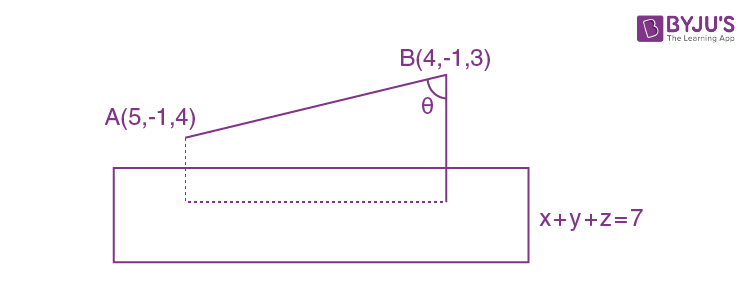Direction ratios of AB = (1,0,1)

Let be angle between line AB and normal of plane.

So cos = 1×1+0×1+1×1)/√2√3

= √2/√3

So projection of line AB =

$$\begin{array}{l}|\vec{AB}|sin \theta\end{array}$$

= √2×√(1-2/3)

= √(2/3)

27. PQR is a triangular park with PQ = PR = 200 m. A T.V. tower stands at the mid-point of QR. If the angles of elevation of the top of the tower at P, Q and R are respectively 450, 300 and 300, then the height of the tower (in m) is :
a) 100√3
b) 50√2
c) 100
d) 50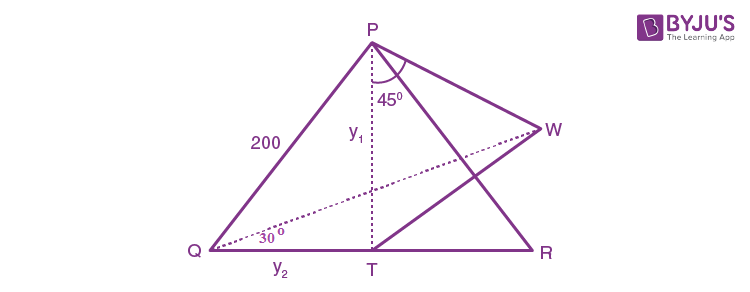Let TW = h

ΔPTW, tan 450 = h/y1

h = y1

Similarly, h/y2 = tan 300

y2 = √3h

ΔPQT, PQ2 = QT2+PT2

40000 = 3h2+h2

4h2 = 40000

h = 100

28. From 6 different novels and 3 different dictionaries, 4 novels and 1 dictionary are to be selected and arranged in a row on a shelf so that the dictionary is always in the middle. The number of such arrangements is :
a) at least 500 but less than 750
b) at least 750 but less than 1000
c) at least 1000
d) less than 500

We have 6 novels and 3 dictionaries. We can select 4 novels and 1 dictionary in

6C4 ×3C1 = 6!×3/(4!2!)

= 6×5×3/2

= 45 ways.

Now 4 novels and 1 dictionary are to be arranged so that dictionary is always in middle. So remaining 4 novels can be arranged in 4! ways.

Hence total arrangements possible are

45×24 = 1080 ways

29. Let A be the sum of the first 20 terms and B be the sum of the first 40 terms of the series.

12 +2.22+32+2.42+52 + 2.62+..

If B-2A = 100λ, then λ is equal to :
a) 464
b) 496
c) 232
d) 248

B = (12+32+52+…+392)+2(22+42+…+402)

= (12+22+32+…+402)+(22+42+…+402)

= (12+22+32+…+402)+4(12+22+32+…+202)

A = (12+22+32+…+202)+4(12+22+32+…+102)

Using 12+22+..n2 = n(n+1)(2n+1)/6

B-2A = 24800

Hence λ = 248

30. Let the orthocentre and centroid of a triangle be A(-3, 5) and B(3, 3) respectively. If C is the circumcentre of this triangle, then the radius of the circle having line segment AC as diameter, is:
a) 3√(5/2)
b) 3√5/2
c) √10
d) 2√10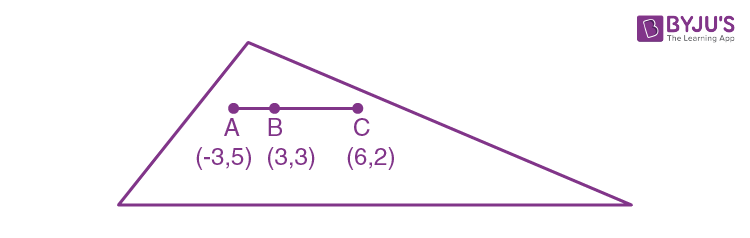As we know centroid divides line joining circumcentre and orthocentre internally 1:2.

So, C(6,2)

AC = √[(6+3)3+(2-5)2]

= √90

= 3√10

r = AC/2

= 3√10/2

= 3√(5/2)